# CBSE Class 10 Physics Magnetic Effects Of Electric Current Worksheet Set A

Read and download free pdf of CBSE Class 10 Physics Magnetic Effects Of Electric Current Worksheet Set A. Students and teachers of Class 10 Science can get free printable Worksheets for Class 10 Science Chapter 13 Magnetic Effect of Electric Current in PDF format prepared as per the latest syllabus and examination pattern in your schools. Class 10 students should practice questions and answers given here for Science in Class 10 which will help them to improve your knowledge of all important chapters and its topics. Students should also download free pdf of Class 10 Science Worksheets prepared by school teachers as per the latest NCERT, CBSE, KVS books and syllabus issued this academic year and solve important problems with solutions on daily basis to get more score in school exams and tests

## Worksheet for Class 10 Science Chapter 13 Magnetic Effect of Electric Current

Class 10 Science students should refer to the following printable worksheet in Pdf for Chapter 13 Magnetic Effect of Electric Current in Class 10. This test paper with questions and answers for Class 10 will be very useful for exams and help you to score good marks

### Class 10 Science Worksheet for Chapter 13 Magnetic Effect of Electric Current

MAGNETIC EFFECTS OF ELECTRIC CURRENT

INTRODUCTION:

We use many appliances at home, like the mixers, grinders, fans etc which draw electricity and convert them to motor movement i.e., mechanical energy. Also, we know of turbines, windmills, generators etc which move a mechanical part to generate electricity. These are possible because when electric current flows through a wire, it produces a magnetic effect around it. So, in this chapter, we will study about these interesting facts -'Magnetic effects of Electric Current'Magnetic Field around a Bar Magnet
• A magnet always exerts an influence around the region surrounding it. This region is called the Magnetic Field.
• Magnetic Field has both direction and quantity.
• The fields always emerge out of the North pole and always merge into the South pole
• Inside the magnet, the field is from the south pole to the north pole, ie merge into the south pole and emerge out of the north pole.• The strength of the magnetic field is determined by the closeness of the field lines.
• If the lines are closer and crowded, it means that the strength of the magnetic field is high and exerts a strong force on a magnet which is brought near it.
• If the lines are far and less crowded, it means that the strength of the magnetic field is relatively low and exerts a weaker force on a magnet which is brought in its proximity.
• When a magnetic compass is brought near a bar magnet, it deflects and always points in the north-south direction.
• When iron filings are brought in the vicinity of a bar magnet, they arrange themselves along the field lines.
• The magnetic field lines are such that they never cross each other. If they did cross at a certain point, it means that at that point, the compass needle would point towards two directions, which is logically incorrect.

Magnetic Field in a Straight Current Carrying conductor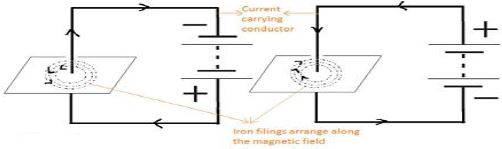• In the above figures, note that there are current carrying conductors in opposite directions. In both cases, the current carrying conductor is intercepted by a cardboard placed at right angles to the current carrying conductor.
• There are some iron fillings sprinkled on the conductor.
• When current flows through the conductor, the iron filing arrange themselves along the magnetic field.
• We can see that the magnetic field in both cases is in opposite directions as is the current.
• The magnetic field produced by a current-carrying straight wire depends inversely on the distance from it and directly on the current passing through it.
• From this we see that the current carrying conductor produces a magnetic field around it. The direction of this magnetic field is given by Right Hand Thumb Rule.

Right Hand Thumb RuleSuppose that you are holding a current-carrying straight conductor in your right hand such that the thumb points towards the direction of flow of current. Then, your fingers which wrap around the conductor indicate the direction of magnetic field lines (as shown in the figure)

Magnetic Field in a Circular Carrying conductor• At every point of current-carrying circular conductor, the magnetic field is in the form of concentric circles as represented above.
• The circles are concentric in nature with increasing diameters as the move farther from the current carrying wire.
• At the centre of the circular loop, the circles appear like straight lines.
• The magnetic field produced by a current-carrying straight wire depends inversely on the distance from it and directly on the current passing through it.
The Right-Hand Thumb Rule is applicable here at every point of the current carrying conductor

Magnetic Field due to Current in a SolenoidA coil with many circular close turns of insulated copper wire (like a cylinder as shown above) is a solenoid.
One end of such a solenoid behaves like the north pole and the other as a south pole.
Therefore, magnetic field due to current in the solenoid is similar to a bar magnet. The fields always emerge out of the North pole and always merge into the South pole
The field inside the solenoid is uniform.
The strong magnetic field inside the solenoid is so strong that it can be used to magnetize a piece of soft iron when it is placed inside the coil. The magnet formed like this is called a Electromagnet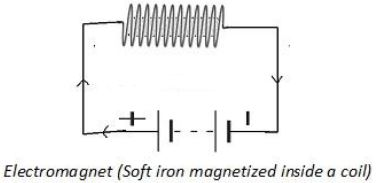Force acting on a Current carrying conductor
An electric current flowing through a conductor produces a magnetic field. This field will exert a force on a magnet placed in the proximity of the conductor.
This means that the magnet also will exert an equal and opposite force on the current-carrying conductor.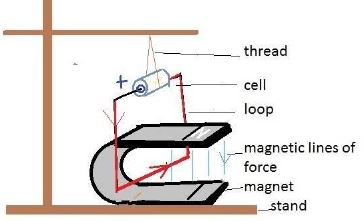● André-Marie Ampère was a French physicist and mathematician who was one of the founders of the science of classical electromagnetism, he discovered that a wire carrying electric current can attract or repel another wire next to it that also carrying electric current.

● When a current carrying suspended conductor placed in a magnetic field it experiences a force in a particular direction. When the direction of the current is reversed the force exerted also changes
the direction.
The direction of this force is given by Left Hand Thumb Rule

- Fleming’s Left Hand rule: Stretch the thumb, forefinger and middle finger of your left hand such that they are mutually perpendicular to each other (as shown in the figure). If the middle finger points in the direction of the current in the conductor, the forefinger points in the direction of the magnetic field and the thumb points in the direction of the force acting on the conductor.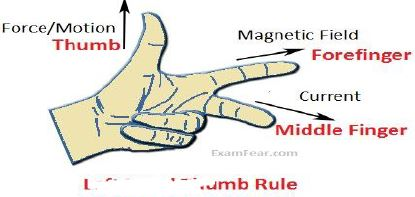Devices that use these applications is electric motor, electric generator, loudspeakers, microphones and measuring instruments.

Electric Motor: A device that converts electrical energy into mechanical energy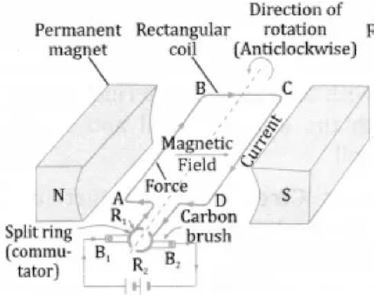Construction of Electric Motor
(a) Armature coil: It consist of a single loop of an insulated copper wire in the form of a rectangle. (b) Strong field magnet; Armature coil is placed between 2 pole pieces of a strong magnet which provide strong magnetic field.
(c) Split ring type commutator: It consist of 2 halves of a metallic ring. The two ends of armature coil are connected to these 2 halves of ring. Commutator reverses the direction of current in armature coil.
(d) Brushes; Two carbon brushes press against the commutator. These brushes act as contact between commutator and terminal battery.
(e) Battery; It is connected across the carbon brushes. It supplies current to the armature coil.

Working of Electric Motor
1)1n the side AB of the rectangular coil ABCD, the direction of current is from A to B and in the side CD of the coil, the direction of current is from C to D. The direction of magnetic field is from N pole of the magnet to its S pole.
2) Applying Fleming’s Left hand rule to sides AB and CD of the coil, the force on side AB of the coil is in downward direction whereas the force on side CD of the coil is in upward direction. Due to this the side AB of the coil is pushed down and side CD is pushed up. This makes the coil ABCD rotate in the anticlockwise direction.
3) While rotating, when the coil reached vertical position, then the brushes will touch the gap between the two commutator rings and current of the coil is cut off. Though the current to the coil is cut off when it is in the exact vertical position, the coil does not stop rotating because it has already gained momentum due to which it goes beyond the vertical position.
4) After half rotation, when the coil goes beyond vertical position, the side CD of the coil comes on the left side whereas side AB of the coil comes to the right side, and the two commutator half rings automatically change contact from one brush to the other.
5) After half rotation of the coil , the commutator half ring R2 makes contact with brush B1 whereas the commutator half ring R1 makes contact with brush B2.This reverse the direction of current in the coil.
6) The reversal of direction of current reverses the direction of force acting on the sides AB and CD of the coil. The side CD of the coil is now on the left side with a downward force on it whereas the side AB is now on the right side with an upward force on it due to this the side CD of the coil is pushed down and the side AB of coil is pushed up. This makes the coil rotate anticlockwise by another half rotation.
7) The reversing of current in the coil is repeated after every half rotation due to which the coil continue to rotate as long as current from the battery is passed through it

Uses of D.C. Motor
It is used in electric cars, rolling mills, electric cranes, lifts, drilling machine, fan, hair dryers, blowers, tape recorder, refrigerators, washing machine, mixers, blenders etc.

AC current (Alternating current) : Changes its direction after equal intervals of time. It is easier to transmit this current over long distances due to lesser loses and hence this is the current that is supplied to our houses from the electricity department.

DC current (Direct current): Does not change direction with time. Eg: Current from a simple battery/cell

Domestic Electric circuits: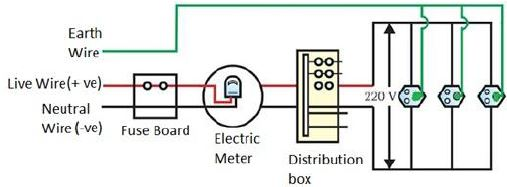We receive power in our house through a main supply, commonly called mains. It is supplied through overhead cables or underground cables.
There are 3 types of wires in domestic circuits: Earth Wire, Live Wire, Neutral Wire.

Earth wire: It is generally green in colour. It is usually connected to a metal plate placed in the earth near the house as a safety measure to ground gadgets that have a metallic body. (Refrigerator, toaster). In case of charges leaking on to the metallic body, the charges get grounded and thus prevent shocks.

Live wire: Positive wire generally red in colour

Neutral wire: Negative wire generally black in colour

The potential difference (or voltage) supplied in our country is 220V
When they come into our houses, they pass through a circuit called a Fuse. Whenever there is a high voltage, voltage fluctuation, overloading, short circuit the fuse melts and prevents the high voltage reaching the electric appliance. This saves the electrical gadget.
Then through the metre board in the house, these wires pass on to different electric gadgets
Generally, 2 types of electric circuits are used at homes

15A: Appliances which have higher power ratings. (Geysers, refrigerators, ACs)

5A: Appliances which have lower power ratings. (TV, bulbs, fans)

MCQS

Question : Which of the following correctly describes the magnetic field near a long straight wire?
(a) The field consists of straight lines perpendicular to the wire.
(b) The field consists of straight lines parallel to the wire.
(c) The field consists of radial lines originating from the wire.
(d) The field consists of concentric circles centred on the wire.

Question : The phenomenon of electromagnetic induction is
(a) the process of charging a body.
(b) the process of generating magnetic field due to a current passing through a coil.
(c) producing induced current in a coil due to relative motion between a magnet and the coil.
(d) the process of rotating a coil of an electric motor.

Question : The device used for producing electric current is called a
(a) generator.
(b) galvanometer.
(c) ammeter.
(d) motor.

Question : The essential difference between an AC generator and a DC generator is that
(a) AC generator has an electromagnet while a DC generator has permanent magnet.
(b) DC generator will generate a higher voltage.
(c) AC generator will generate a higher voltage.
(d) AC generator has slip rings while the DC generator has a commutator.

Question : At the time of short circuit, the current in the circuit.
(a) reduces substantially.
(b) does not change.
(c) increases heavily.
(d) vary continuously.

Question : The rating of a fuse connected in the lighting circuit is __________.
(a) 5 A
(b) 15 A
(c) 0 A
(d) 10 A

Question : The core of electromagnet is:
(a)Soft iron
(b) Nickel
(c) copper
(d) Steel

Question : An electric charge in uniform motion produces:
(a) an electric field only
(b) a magnetic field only
(c) both electric and magnetic fields
(d) both electric and magnetic fields

Question : The magnetic field lines always begin from
(a) N-pole and end on S-pole.
(b) S-pole and end on N-pole.
(c) start from the middle and end at N-pole.
(d) start from the middle and end at S-pole.

Question : The magnetic field is the strongest at
(a) middle of the magnet.
(b) north pole.
(c) south pole.
(d) both poles

Question : A soft iron bar is introduced inside a current carrying solenoid. The magnetic field inside the solenoid
(a) will become zero.
(b) will increase.
(c) will decrease.
(d) will remain unaffected

Question : A magnetic field directed in north direction acts on an electron moving in east direction.
The magnetic force on the electron will act
(a) vertically upwards.
(b) towards east.
(c) vertically downwards.
(d) towards north

Question : The direction of force on a current carrying conductor in a magnetic field is given by
(a) Fleming’s left-hand rule.
(b) Fleming’s right-hand rule.
(c) Right hand thumb rule.
(d) Left hand thumb rule.

Question : Switches are connected to
(a) live wire.
(b) neutral wire.
(c) earth wire.
(d) anyone.

Question : The most important safety method used for protecting home appliances from shortcircuiting or Overloading is
(a) earthing
(b) use of stabilizers
(c) use of fuse
(d) use of electric meter

Question : A circular loop placed in a plane perpendicular to the plane of paper carries a current when the keys is ON. The current as seen from points A and B (in the plane of paper and on the axis of the coil) is anticlockwise and clockwise respectively. The magnetic field lines point from B to A. The N-pole of the resultant magnet is on the faces close to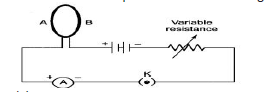(a) A
(b) B
(c) A if the current is small, and B if the current is large
(d) B if the current is small and A if the current is large.

Question : Choose the incorrect statements from the following regarding magnetic lines of field.
(a) the direction of magnetic field at a point is taken to be the direction in which the north pole of a magnetic compass needle points
(b) magnetic field lines are closed curves
(c) if magnetic field lines are parallel and equidistant, they represent zero field strength
(d) relative strength of magnetic field is shown by the degree of closeness of the field lines.

Question : If the key in the arrangement figure given below is taken out (the circuit is made open) and magnetic. field lines are drawn over the horizontal plane ABCD, the lines are(a) concentric circles
(b) elliptical in shape
(c) straight lines parallel to each other (Due to earth’s magnetic field)
(d) concentric circles near the point O but of elliptical shapes as we go away from it.

Question : A constant current flow in a horizontal wire in the plane of the paper from east to west as shown in the figure. The direction of magnetic field at a point will be North to South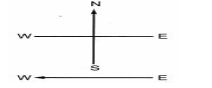(a) directly above the wire
(b) directly below the wire
(c) at a point located in the plane of the paper, on the north side of the wire
(d) at a point located in the plane of the paper, on the south side of the wire

Question : In the arrangement shown in the figure there are two coils wound on a non-conducting cylindrical rod. Initially the key is not inserted. Then the key is inserted and later removed.
Then(a) the deflection in the galvanometer remains zero throughout.
(b) there is a momentary deflection in the galvanometer but it dies out shortly and there is no effect when the keys are removed.
(c) there are momentary galvanometer deflections that die out shortly; the deflections are in the same direction.
(d) there are momentary galvanometer deflections that die out shortly; the deflection are in opposite directions.

Assertion-Reason Type Questions

For question numbers 6 and 10, two statements are given-one labelled as Assertion
(A) and the other labelled Reason (R). Select the correct answer to these questions from the codes (a), (b), (c) and (d) as given below:
(a) Both ‘A’ and ‘R’ are true and ‘R’ is correct explanation of the Assertion.
(b) Both ‘A’ and ‘R’ are true but ‘R’ is not correct explanation of the Assertion.
(c) ‘A’ is true but ‘R’ is false.
(d) ‘A’ is false but ‘R’ is true.

Question : Assertion: Magnetic field lines do not intersect.
Reason: Magnetic field lines are closed curves

Question : Assertion: A compass needle gets defected when electric current is passed through the nearly metallic wire.
Reason: The S.I unit of magnetic field strength is Oersted

Question : Assertion: A current carrying solenoid behaves like a bar magnet.
Reason: When soft iron is placed inside the solenoid it can also be magnetised.

Question : Assertion: Compass is a small magnet and gives direction of magnetic field lines.
Reason: It gets deflected when brought near a bar magnet.

Question :  Assertion: Galvanometer is used to detect current.
Reason: Ammeter is used measure current generated in A.C. generator

Question : Why does a compass needle get deflected when brought near a bar magnet?
Answer- A compass needle is a small bar magnet. When it is brought near a bar magnet, its magnetic field lines interact with that of the bar magnet. Hence, a compass needle shows a deflection when brought near the bar magnet.

Question : Draw magnetic field lines around a bar magnet.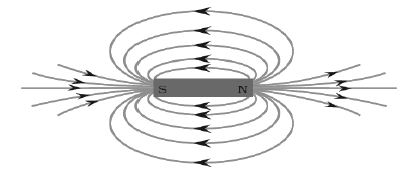Question : List the properties of magnetic lines of force.
Answer- (a) The direction of field lines outside the magnet is from the North Pole to the South Pole.
(b) The direction of field lines inside the magnet is from the South Pole to the North Pole.
(c) Relative density of magnetic field lines tells about the strength of magnetic field.
(d) Magnetic lines do not intersect with each other.

Question : Why don’t two magnetic lines of force intersect each other?
Answer- If two field lines of a magnet intersect, then at the point of intersection, the compass needle points in two different directions. This is not possible. Hence, two field lines do not intersect each other.

Question 5: Consider a circular loop of wire lying in the plane of the table. Let the current pass through the loop clockwise. Apply the right-hand thumb rule to find out the direction of the magnetic field inside and outside the loop.
Answer- Inside the loop = Pierce inside the table, Outside the loop = Appear to emerge out from the table.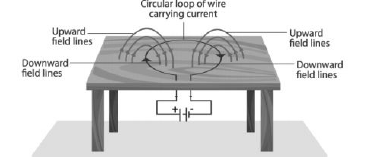Question : The magnetic field in a given region is uniform. Draw a diagram to represent it.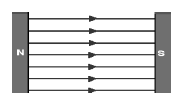Question : Choose the correct option. The magnetic field inside a long straight solenoid-carrying current:
(a) is zero
(b) decreases as we move towards its end
(c) increases as we move towards its end
(d) is the same at all points

Question : In Activity 13.7, how do we think the displacement of rod AB will be affected if (i) current in rod AB is increased; (ii) a stronger horse-shoe magnet is used; and (iii) length of the rod AB is increased?
Answer- Displacement of rod AB will increase in all the three cases, because force experienced by a conductor placed in magnetic field is directly proportional to current, Length of conductor and strength of Magnetic field. { F = ILB }

Question : A positively-charged particle (alpha-particle) projected towards west is deflected towards north by a magnetic field. The direction of magnetic field is
(a). towards south
(b). towards east
(c). downward
(d). upward ✓

Question : Which of the following property of a proton can change while it moves freely in a magnetic field? (There may be more than one correct answer.)
(a) mass
(b) speed
(c) velocity ✓
(d) momentum✓

Question : State Fleming’s left-hand rule.
Answer- Fleming’s left hand rule states that if we arrange the thumb, the middle finger, and the forefinger of the left hand at right angles to each other, if the forefinger point towards the direction of the magnetic field, the middle finger points in the direction of current, then the thumb gives the direction of force or motion.

Question : What is the principle of an electric motor?
Answer- A current-carrying loop experiences a torque (rotating force) force and rotates when placed in a magnetic field. The direction of rotation of the loop is given by the Fleming’s left-hand rule.

Question : What is the role of the split ring in an electric motor?
Answer- The split ring in the electric motor acts as a commutator. The commutator reverses the direction of current flowing through the coil after each half rotation of the coil. Due to this reversal of the current, the coil continues to rotate in the same direction.

Question : Explain different ways to induce current in a coil.
Answer- (a) If a coil is moved rapidly between the two poles of a horse-shoe magnet, then an electric current is induced in the coil.
(b) If a magnet is moved relative to a coil, then an electric current is induced in the coil.

Question : State the principle of an electric generator.
Answer- An electric generator works on the principle of electromagnetic induction. It states that whenever there is a change in magnetic flux of a coil, an emf or current is induced in the coil.

Question : Name some sources of direct current.
Answer- Some sources of direct current are cell, DC generator, etc.

Question : Which sources produce alternating current?
Answer- AC generators, power plants, etc., produce alternating current.

Question : Choose the correct option: A rectangular coil of copper wires is rotated in a magnetic field.The direction of the induced current changes once in each:
(a) two revolutions
(b) one revolution
(c) half revolution
(d)one-fourth revolution

Question : Name two safety measures commonly used in electric circuits and appliances.

Question : An electric oven of 2 kW is operated in a domestic electric circuit (220 V) that has a current rating of 5 A. What result do you expect? Explain.
Answer- Power = 2 kW = 2000 W
Potential difference = V = 220 V
Current drawn by oven = I =p/v=2000/200 = 9.09 A
As oven draws current 9.09 A but safety limit (rating) of current in the circuit is 5 A, So fuse will melt and circuit will break.

Question : What precaution should be taken to avoid the overloading of domestic electric circuits?
Answer- (a) Too many appliances should not be connected to a single socket.
(b) Too many appliances should not be used at the same time.
(c) Faulty appliances should not be connected in the circuit.
(d) Fuse should be connected in the circuit.

Question : Which of the following correctly describes the magnetic field near a long straight wire?
(a) The field consists of straight lines perpendicular to the wire
(b) The field consists of straight lines parallel to the wire
(c) The field consists of radial lines originating from the wire
(d) The field consists of concentric circles centred on the wire ✓

Question : The phenomenon of electromagnetic induction is:
(a) the process of charging a body
(b) the process of generating magnetic field due to a current passing through a coil
(c) producing induced current in a coil due to relative motion between a magnet and the coil ✓
(d) the process of rotating a coil of an electric motor.

Question : The device used for producing electric current is called a:
(a) generator
(b) galvanometer
(c) ammeter
(d) motor.

Question : The essential difference between an AC generator and a DC generator is that:
(a) AC generator has an electromagnet while a DC generator has permanent magnet.
(b) DC generator will generate a higher voltage.
(c) AC generator will generate a higher voltage.
(d) AC generator has slip rings while the DC generator has a commutator. ✓

Question : At the time of short circuit, the current in the circuit:
(a) reduces substantially
(b) does not change
(c) increases heavily
(d) vary continuously.

Question : State whether the following statements are true or false.
(a) An electric motor converts mechanical energy into electrical energy - FALSE
(b) An electric generator works on the principle of electromagnetic induction -TRUE
(c) The field at the centre of a long circular coil carrying current will be parallel straight lines -TRUE
(d) A wire with a green insulation is usually the live wire of an electric supply –FALSE.

Question : List three sources of magnetic fields
Answer- Three sources of magnetic fields are as follows:
(a) Current-carrying conductors
(b) Permanent magnets
(c) Electromagnets.

Question : How does a solenoid behave like a magnet? Can you determine the north and south poles of a current-carrying solenoid with the help of a bar magnet? Explain.
Answer- A solenoid is a long coil of circular loops of insulated copper wire. Magnetic field lines are produced around the solenoid when a current is allowed to flow through it.
The magnetic field produced by it is similar to the magnetic field of a bar magnet. End of solenoid having current in clockwise direction behaves as South pole, so it will attract North pole of a bar magnet. On the other hand the end of solenoid having current in anticlockwise direction behaves as North pole, so it will repel the North pole of a bar magnet.

Question : When is the force experienced by a current-carrying conductor placed in a magnetic field largest?
Answer- When the direction of current is perpendicular to the direction of the magnetic field.

Question : Imagine that you are sitting in a chamber with your back to one wall. An electron beam, moving horizontally from back wall towards the front wall, is deflected by a strong magnetic field to your right side. What is the direction of magnetic field?
Answer- By Fleming’s left hand rule magnetic field inside the chamber will be downward.

Question : Draw a labelled diagram of an electric motor. Explain its principle and working. What is the function of a split ring in an electric motor?
Answer- An electric motor converts electrical energy into mechanical energy. It works on the principle of the magnetic effect of current. A current-carrying coil rotates in a magnetic field. Direction of rotation can be determined by using Fleming’s left hand rule. The following figure shows a simple electric motor.Working- When current is made to flow through the coil ABCD by closing the switch, the coil starts to rotate in the anticlockwise direction. This is due to the downward force acting on the length AB and simultaneously upward force acts along the length CD. As a result of which the coil rotates in the anticlockwise direction.
Function of split ring- The split ring in the electric motor acts as a commutator. The commutator reverses the direction of current flowing through the coil after each half rotation of the coil. Due to this reversal of the current, the coil continues to rotate in the same direction.

Question : Name some devices in which electric motors are used?
Answer- (a) Water pumps (b) Electric fans (c) Electric mixers (d) Washing machines etc.

Question : A coil of insulated copper wire is connected to a galvanometer. What will happen if a bar magnet is (i) pushed into the coil, (ii) withdrawn from inside the coil, (iii) held stationary inside the coil?
Answer- (i) When a bar magnet is pushed into the coil, current is induced in the coil momentarily as a result the galvanometer deflects in a particular direction momentarily.
(ii) When the bar magnet is withdrawn from inside the coil, current is induced momentarily but in the opposite direction and the galvanometer deflects in the opposite direction momentarily.
(iii) When the bar magnet is held stationary inside the coil, no current will be induced as a result there will be no deflection in the galvanometer.

Question : Two circular coils A and B are placed closed to each other. If the current in the coil A is changed, will some current be induced in the coil B? Give reason.
Answer- Yes, when the current in coil A is changed, the magnetic field associated with it also changes. As a result, the magnetic field around coil B also changes. This change in magnetic field lines around coil B induces an electric current in it.

Question : State the rule to determine the direction of a:
(i) Magnetic field produced around a straight conductor-carrying current.
Answer- Maxwell’s right hand thumb rule. It states that imagine you are holding a current-carrying wire in your right hand such that your thumb points in direction of the current, then the direction in which fingers wrap the wire represents the direction of magnetic lines of force.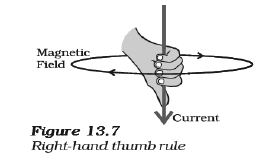(ii) Force experienced by a current-carrying straight conductor placed in a magnetic field which is perpendicular to it.
Answer- Fleming’s left hand rule. Fleming’s left hand rule states that if we arrange the thumb, the middle finger, and the forefinger of the left hand at right angles to each other, if the forefinger point towards the direction of the magnetic field, the middle finger points in the direction of current, then the thumb gives the direction of force or motion.(iii) Current induced in a coil due to its rotation in a magnetic field.
Answer- Fleming’s right hand rule. It states that if we arrange the thumb, fore finger and middle finger of the right hand at right angles to each other. Then if the forefinger represents the direction of the magnetic field, the thumb points in the direction of motion or applied force, then the middle finger points in the direction of the induced current.

Question : Explain the underlying principle and working of an electric generator by drawing a labelled diagram. What is the function of brushes?
Answer- An electric generator converts mechanical energy into electrical energy. An electric generator works on the principle of electromagnetic induction. It states that whenever there is a change in magnetic flux of a coil, an emf or current is induced in the coil. The following figure shows a simple AC generator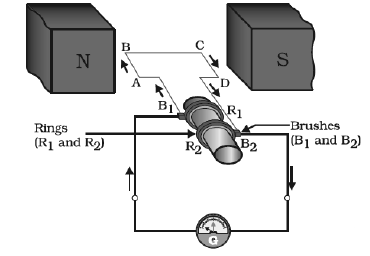When the axle is rotated clockwise, AB moves upwards while CD moves downward. Therefore, according to Fleming’s right hand rule, the direction of the induced current will be from A to B along the length AB. Similarly, the direction of the induced current will be from C to D along the length CD. Hence, galvanometer shows a deflection in a particular direction. After half a rotation, length AB starts moving downwards while the length CD starts moving upwards. Now, the direction of the induced current reverses to DCBA. Since the direction of the induced current reverses every half rotation, the current induced is known as alternating current. Function of Brushes- Brushes help in transferring current from coil to the external circuit.

Question : When does an electric short circuit occur?
Answer- When the insulation of live and neutral wires undergoes wear and tear and then touches each other, the current flowing in the circuit increases abruptly. Hence, a short circuit occurs.

Question : What is the function of an earth wire? Why is it necessary to earth metallic appliances?
Answer- The metallic body of electric appliances is connected to the earth by means of earth wire so that any leakage of electric current is transferred to the ground. This prevents any electric shock to the user. That is why earthing of the electrical appliances is necessary.

More Question-

Question. Why cannot two magnetic field lines intersect?
Ans. Two magnetic field lines never intersect because the resultant force on a north pole at any point can only be in one direction. But if two magnetic field lines intersect one another,
then the resultant force on a north pole placed at the point of intersection will be along two directions, which is not possible.

Question. List the properties of magnetic field lines?
Ans. Properties of magnetic field lines:
(1) Magnetic field is a quantity that has both direction and magnitude.
(2) They emerge from the north pole of a magnet and enter at the south pole of the magnet.
(3) Inside the magnet, the direction of field lines is from south pole to its north pole. Hence, magnetic field lines are closed curves.
(4) The relative strength of the magnetic field is shown by the degree of closeness of the field lines. Crowded field lines represent the strong magnetic field.
(5) The magnetic field at any point is represented by the tangent at that point. No two field lines intersect each other. If they intersect, it would mean that at point of intersection there would be two field directions, which is not possible.
(6) They are dense close to the poles and sparse away from them. It means magnetic field is strongest around poles of the magnet.

Question. What is indicated by crowding of magnetic field lines in a given region?
Ans. The crowding of magnetic field lines in a given region indicates that the magnetic field is stronger in that region.

Question. Why do commercial motors use a soft iron core on which the coil is wound?
Ans. Commercial motors use a soft iron core on which the coil is wound to increase the power of the electric motor.

Question. Why is current induced in the secondary coil when current is changed in the primary coil?
Ans. Current is induced in the secondary coil as the magnetic field lines associated with it are changing due to change in current in the primary coil resulting in change in magnetic
field associated with the primary coil

Question. Which of the following correctly describes the magnetic field near a long straight wire?
a. The field consists of straight lines perpendicular to the wire.
b. The field consists of straight lines parallel to the wire.
c. The field consists of radial lines originating from the wire.
d. The field consists of concentric circles centred on the wire.
Answer. d. The field consists of concentric circles centred on the wire.
The magnetic field near a long straight wire are concentric circles. Their centers lie on the wire.

Question. The phenomenon of electromagnetic induction is
a. the process of charging a body.
b. the process of generating magnetic field due to a current passing through a coil.
c. producing induced current in a coil due to relative motion between a magnet and the coil.
d. the process of rotating a coil of an electric motor.
Answer. c. producing induced current in a coil due to relative motion between a magnet and the coil.
The phenomenon of inducing current in a coil due to the relative motion between the coil and the magnet
Is known as electromagnetic induction.

Question. The device used for producing electric current is called a
a.generator
b. galvanometer
c. ammeter
d. motor
The device used for producing electric current is known as generator. Generator converts mechanical energy to electric energy.

Question. The essential difference between an AC generator and a DC generator is that
a. AC generator has an electromagnet while a DC generator has permanent magnet.
b. DC generator will generate a higher voltage.
c. AC generator will generate a higher voltage.
d. AC generator has slip rings while the DC generator has a commutator.
Answer. d. AC generator has slip rings while the DC generator has a commutator.
AC generators have two rings known as the slip rings while DC generators have two half rings known as the commutator. This is main difference between AC generator and DC generator.

Question. At the time of short circuit, the current in the circuit
a. reduces substantially.
b. does not change.
c. increases heavily.
d. vary continuously.
When two naked wires in the circuit come in contact with each other, the amount of current flowing in the circuit increase abruptly resulting in short circuit.

Question. State whether the following statements are true or false.
a. An electric motor converts mechanical energy into electrical energy.
b. An electric generator works on the principle of electromagnetic induction.
c. The field at the center of a long circular coil carrying current will be parallel straight lines.
d. A wire with a green insulation is usually the live wire of an electric supply.
a. False
An electric motor converts electrical energy into mechanical energy.
b. True
An electric generator is a device that generates electricity by rotating a coil in a magnetic field.
c. True
A long circular coil is a solenoid. The magnetic field lines inside a solenoid are parallel straight lines.
d. False
Live wires have red insulation cover while the earth wire has green insulation.

Question. List two methods of producing magnetic fields.
Following are the methods of producing magnetic fields:
By using a permanent magnet we can produce magnetic field and it can be visualized by spreading iron fillings on a white paper and keeping a magnet beneath the paper.
A current carrying straight conductor produces magnetic field.
Different types of conductors such as solenoid and circular loop can be used to see the presence of magnetic field.

Question. How does a solenoid behave like a magnet? Can you determine the north and south poles of a current–carrying solenoid with the help of a bar magnet? Explain.
A solenoid is a long coil of circular loops of insulated copper wire. The magnetic field produced around the solenoid when the current is passed through it is similar to the magnetic field produced around the bar magnet when current is passed through it. The figure shown below shows the arrangement of magnetic fields produced around the solenoid when current is passed through it.When the north pole of the bar magnet is brought close to the end connected to the negative terminal of the battery, the solenoid repels the battery. As like poles repel each other, we can infer that the end connected to the negative terminal behaves as a north pole while the end connected to the positive terminal behaves as a south pole.

Question. When is the force experienced by a current–carrying conductor placed in a magnetic field largest?
Answer. When the direction of the current is perpendicular to the direction of the magnetic field is when the force experienced is the largest.

Question. Imagine that you are sitting in a chamber with your back to one wall. An electron beam, moving horizontally from back wall towards the front wall, is deflected by a strong magnetic field to your right side. What is the direction of magnetic field?
Answer.The direction of the magnetic field can be determined using the Fleming’s Left hand rule. The direction of the magnetic field will be perpendicular to the direction of current and the direction of deflection, i.e., either upward or downward. The direction of the current is from the front wall to the back wall because negatively charged electrons move from the back wall to the front wall. The directed of the magnetic force is rightward. Hence, using Fleming’s left hand rule it can be concluded that the direction of the magnetic field inside the chamber is downward.

## More Study Material

### CBSE Class 10 Science Chapter 13 Magnetic Effect of Electric Current Worksheet

We hope students liked the above worksheet for Chapter 13 Magnetic Effect of Electric Current designed as per the latest syllabus for Class 10 Science released by CBSE. Students of Class 10 should download in Pdf format and practice the questions and solutions given in the above worksheet for Class 10 Science on a daily basis. All the latest worksheets with answers have been developed for Science by referring to the most important and regularly asked topics that the students should learn and practice to get better scores in their class tests and examinations. Studiestoday is the best portal for Class 10 students to get all the latest study material free of cost.

### Worksheet for Science CBSE Class 10 Chapter 13 Magnetic Effect of Electric Current

Expert teachers of studiestoday have referred to the NCERT book for Class 10 Science to develop the Science Class 10 worksheet. If you download the practice worksheet for one chapter daily, you will get higher and better marks in Class 10 exams this year as you will have stronger concepts. Daily questions practice of Science worksheet and its study material will help students to have a stronger understanding of all concepts and also make them experts on all scoring topics. You can easily download and save all revision worksheet for Class 10 Science also from www.studiestoday.com without paying anything in Pdf format. After solving the questions given in the worksheet which have been developed as per the latest course books also refer to the NCERT solutions for Class 10 Science designed by our teachers

#### Chapter 13 Magnetic Effect of Electric Current worksheet Science CBSE Class 10

All worksheets given above for Class 10 Science have been made as per the latest syllabus and books issued for the current academic year. The students of Class 10 can be rest assured that the answers have been also provided by our teachers for all worksheet of Science so that you are able to solve the questions and then compare your answers with the solutions provided by us. We have also provided a lot of MCQ questions for Class 10 Science in the worksheet so that you can solve questions relating to all topics given in each chapter. All study material for Class 10 Science students have been given on studiestoday.

#### Chapter 13 Magnetic Effect of Electric Current CBSE Class 10 Science Worksheet

Regular worksheet practice helps to gain more practice in solving questions to obtain a more comprehensive understanding of Chapter 13 Magnetic Effect of Electric Current concepts. Worksheets play an important role in developing an understanding of Chapter 13 Magnetic Effect of Electric Current in CBSE Class 10. Students can download and save or print all the worksheets, printable assignments, and practice sheets of the above chapter in Class 10 Science in Pdf format from studiestoday. You can print or read them online on your computer or mobile or any other device. After solving these you should also refer to Class 10 Science MCQ Test for the same chapter.

#### Worksheet for CBSE Science Class 10 Chapter 13 Magnetic Effect of Electric Current

CBSE Class 10 Science best textbooks have been used for writing the problems given in the above worksheet. If you have tests coming up then you should revise all concepts relating to Chapter 13 Magnetic Effect of Electric Current and then take out a print of the above worksheet and attempt all problems. We have also provided a lot of other Worksheets for Class 10 Science which you can use to further make yourself better in Science

Where can I download latest CBSE Printable worksheets for Class 10 Science Chapter 13 Magnetic Effect of Electric Current

You can download the CBSE Printable worksheets for Class 10 Science Chapter 13 Magnetic Effect of Electric Current for latest session from StudiesToday.com

Can I download the Printable worksheets of Chapter 13 Magnetic Effect of Electric Current Class 10 Science in Pdf

Yes, you can click on the links above and download Printable worksheets in PDFs for Chapter 13 Magnetic Effect of Electric Current Class 10 for Science

Are the Class 10 Science Chapter 13 Magnetic Effect of Electric Current Printable worksheets available for the latest session

Yes, the Printable worksheets issued for Class 10 Science Chapter 13 Magnetic Effect of Electric Current have been made available here for latest academic session

How can I download the Class 10 Science Chapter 13 Magnetic Effect of Electric Current Printable worksheets

You can easily access the links above and download the Class 10 Printable worksheets Science Chapter 13 Magnetic Effect of Electric Current for each chapter

Is there any charge for the Printable worksheets for Class 10 Science Chapter 13 Magnetic Effect of Electric Current

There is no charge for the Printable worksheets for Class 10 CBSE Science Chapter 13 Magnetic Effect of Electric Current you can download everything free

How can I improve my scores by solving questions given in Printable worksheets in Class 10 Science Chapter 13 Magnetic Effect of Electric Current

Regular revision of practice worksheets given on studiestoday for Class 10 subject Science Chapter 13 Magnetic Effect of Electric Current can help you to score better marks in exams

Are there any websites that offer free test sheets for Class 10 Science Chapter 13 Magnetic Effect of Electric Current

Yes, studiestoday.com provides all latest NCERT Chapter 13 Magnetic Effect of Electric Current Class 10 Science test sheets with answers based on the latest books for the current academic session

Can test papers for Class 10 Science Chapter 13 Magnetic Effect of Electric Current be accessed on mobile devices

Yes, studiestoday provides worksheets in Pdf for Chapter 13 Magnetic Effect of Electric Current Class 10 Science in mobile-friendly format and can be accessed on smartphones and tablets.

Are worksheets for Chapter 13 Magnetic Effect of Electric Current Class 10 Science available in multiple languages

Yes, worksheets for Chapter 13 Magnetic Effect of Electric Current Class 10 Science are available in multiple languages, including English, Hindi